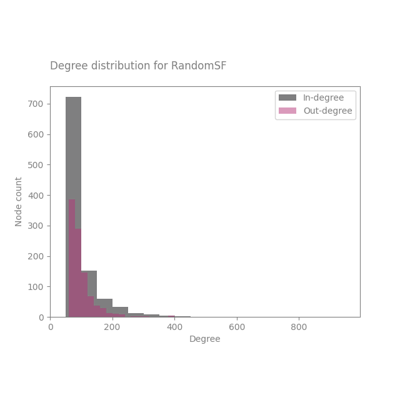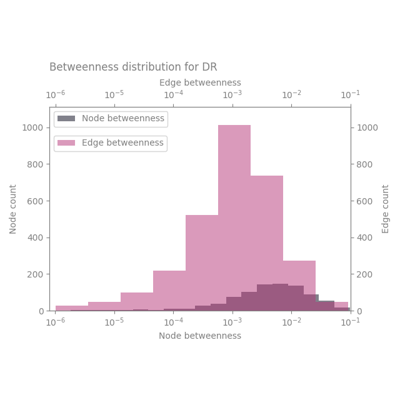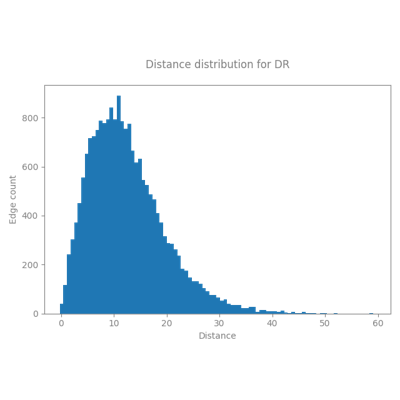# Visualizing graph properties#Plot the degree distributions of a graph

Plot the degree distributions of a graphPlot the betweenness distributions of a graph

Plot the betweenness distributions of a graphPlot various graph properties

Plot various graph properties

Gallery generated by Sphinx-Gallery